7-simplex

﻿
7-simplex
Regular octaexon
(7-simplex)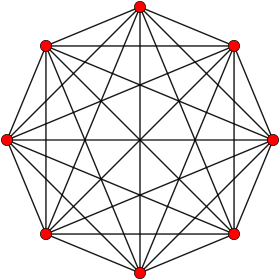Orthogonal projection
inside Petrie polygon
Type Regular 7-polytope
Family simplex
Schläfli symbol {3,3,3,3,3,3}
Coxeter-Dynkin diagram6-faces 8 6-simplex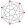5-faces 28 5-simplex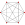4-faces 56 5-cell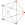Cells 70 tetrahedronFaces 56 triangle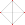Edges 28
Vertices 8
Vertex figure 6-simplex
Petrie polygon octagon
Coxeter group A7 [3,3,3,3,3,3]
Dual Self-dual
Properties convex

In 7-dimensional geometry, a 7-simplex is a self-dual regular 7-polytope. It has 8 vertices, 28 edges, 56 triangle faces, 70 tetrahedral cells, 56 5-cell 5-faces, 28 5-simplex 6-faces, and 8 6-simplex 7-faces. Its dihedral angle is cos−1(1/7), or approximately 81.79°.

Alternate names

It can also be called an octaexon, or octa-7-tope, as an 8-facetted polytope in 7-dimensions. The name octaexon is derived from octa for eight facets in Greek and -ex for having six-dimensional facets, and -on. Jonathan Bowers gives a octaexon the acronym oca.

Coordinates

The Cartesian coordinates of the vertices of an origin-centered regular octaexon having edge length 2 are:$\left(\sqrt{1/28},\ \sqrt{1/21},\ \sqrt{1/15},\ \sqrt{1/10},\ \sqrt{1/6},\ \sqrt{1/3},\ \pm1\right)$$\left(\sqrt{1/28},\ \sqrt{1/21},\ \sqrt{1/15},\ \sqrt{1/10},\ \sqrt{1/6},\ -2\sqrt{1/3},\ 0\right)$$\left(\sqrt{1/28},\ \sqrt{1/21},\ \sqrt{1/15},\ \sqrt{1/10},\ -\sqrt{3/2},\ 0,\ 0\right)$$\left(\sqrt{1/28},\ \sqrt{1/21},\ \sqrt{1/15},\ -2\sqrt{2/5},\ 0,\ 0,\ 0\right)$$\left(\sqrt{1/28},\ \sqrt{1/21},\ -\sqrt{5/3},\ 0,\ 0,\ 0,\ 0\right)$$\left(\sqrt{1/28},\ -\sqrt{12/7},\ 0,\ 0,\ 0,\ 0,\ 0\right)$$\left(-\sqrt{7/4},\ 0,\ 0,\ 0,\ 0,\ 0,\ 0\right)$

More simply, the vertices of the 7-simplex can be positioned in 8-space as permutations of (0,0,0,0,0,0,0,1). This construction is based on facets of the 8-orthoplex.

Images

orthographic projections
Ak Coxeter plane A7 A6 A5
Graph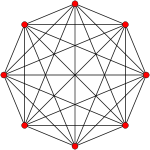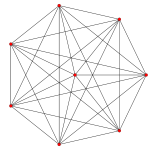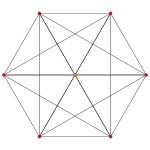Dihedral symmetry   
Ak Coxeter plane A4 A3 A2
Graph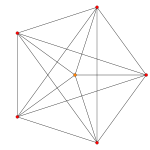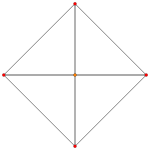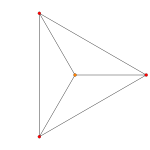Dihedral symmetry   

Related polytopes

This polytope is one of 71 uniform 7-polytopes with A7 symmetry.